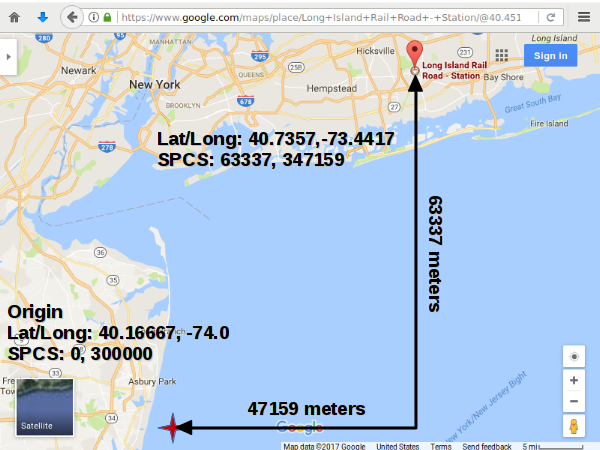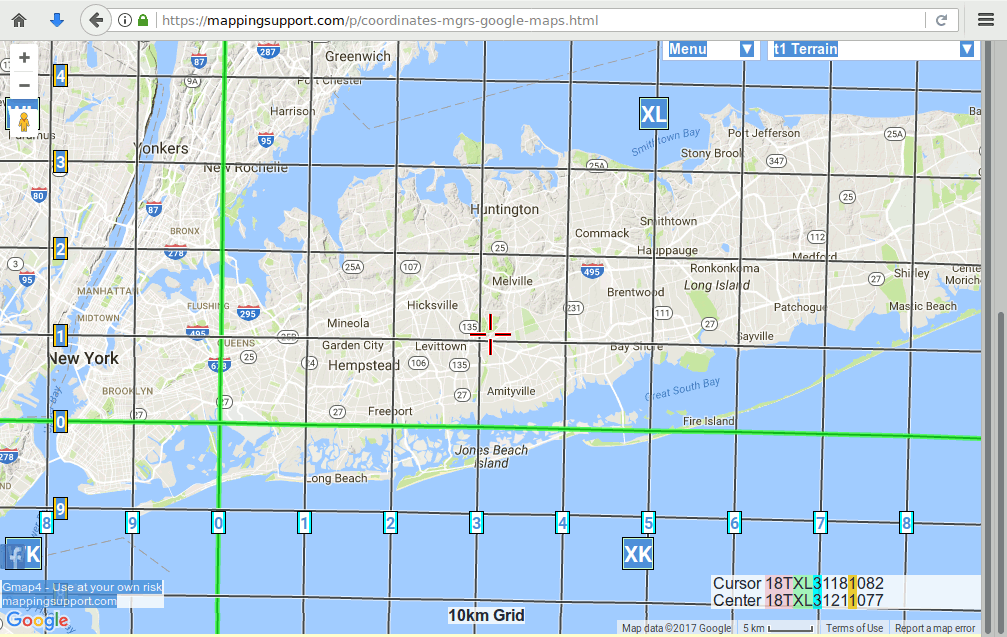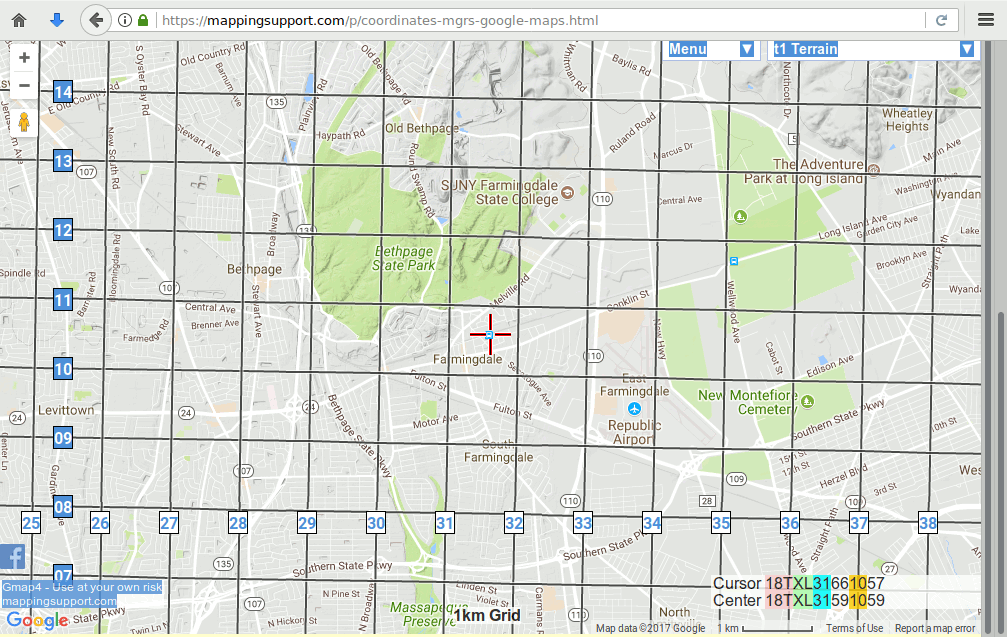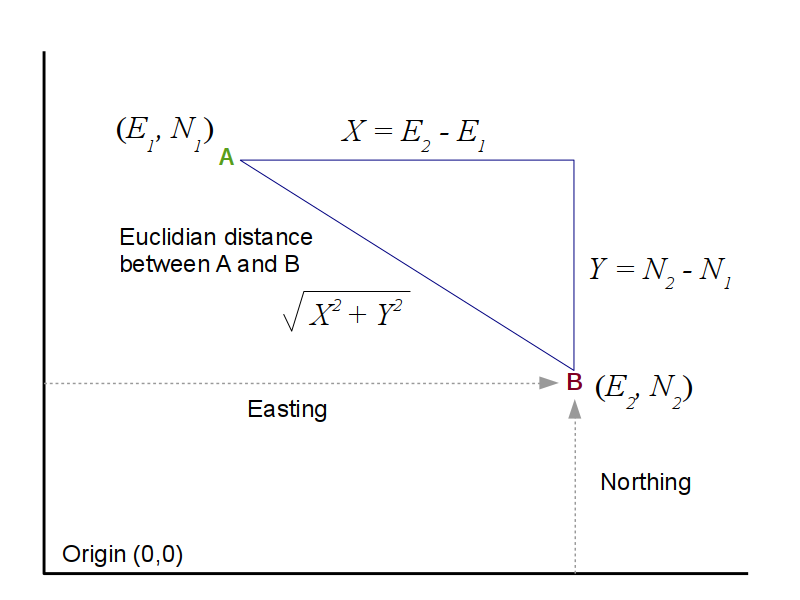# Local Map Projections and Coordinate Systems

The Earth exists in three-dimensions but, other than globes, most representations of the earth are two dimensional. The process of converting a three-dimensional space to a two-dimensional map is called projection.

The process of projection involves distorition of area, distance, shape, and angles. With projections of large areas like the world, these distortions are significant, and dramatic compromises are required depending on the purpose of the map and the aesthetic objectives.

However, on smaller areas of the surface of the earth, our lived experience is as a flat plane. Accordingly, projections can be designed that minimize distortions and reflect that experience. These projections define a coordinate system that represents locations as X and Y coordinates. This provides the ability to accurately find specific locations and measure distances, such as when surveying property lines or engaging in military activity.

While there are hundreds of different projections used around the world for specific areas, three collections of projections are commonly used in the United States:

• The State Plane Coordinate System (SPCS)
• Universal Transverse Mercator (UTM)
• The National Grid / Military Grid Reference System (MGRS)

## The State Plane Coordinate System

The State Plane Coordinate System (SPCS) was developed in the 1930s by the US Coast and Geodetic Survey (predecessor of the National Ocean Service) to enable surveyors, mappers, and engineers to connect their land or engineering surveys to a common reference system. You will commonly see government data distributed with coordinates in SPCS.

There are 124 different zones in different parts of the US, usually aligned to county borders as a convenience.

Zones that are taller than they are wide generally use a transverse Mercator projection.

Zones that are wider than they are tall usually use a Lambert conformal conic projection. The choice of Mercator or conic zones was made to minimize the number of zones per state based on their individual aspect ratio.

The zones are specifically chosen to be small enough that distortion of distance can be greatly minimized to 1 part in 10,000 throughout the zone. This means you can measure a distance of two miles within around a foot of accuracy.

The SPCS projections turn the surface of the earth into a flat plane (hence, the name).

• A latitude of origin is assigned a Y coordinate (northing) of zero meters. It is placed to the south of the zone so all Y coordinates are positive
• A central meridian is placed in the center of the zone
• An origin point with coordinates (0,0) is defined for the zone. This origin point is offset from the central meridian by a false easting and, sometimes, a false northing. This ensures that no X coordinate in the zone is negative. This false easting varies by zone and can vary from 100,000 into the millions
• Coordinates can then be specified in meters east and north of the origin point specific to each zone. These coordinates are called easting and northing to distinguish them from the angular measurements of latitude and longitude

As an example of SPCS coordinates, we use the Farmingdale, NY train station:

• Google Maps gives an estimated WGS84 latitude and longitude of 40.735677, -73.441718
• That can also be expressed in degrees-minutes-seconds as: 40° 44' 8.44" North, 73° 26' 30.2" West
• Using a map of State Plane Coordinate System zones, we see this area is in New York Long Island SPCS zone (FIPS: 3104)
• Using a convertor like this one from the National Geodetic Survey with the New York Long Island zone gives us a northing and easting of 63337 x 347159 meters
• Looking up New York Long Island in spatialreference.org we find the EPSG code for the NAD83 variant is 32118
• Viewing the Well Known Text as HTML we see that:
• The projection is a Lambert Conformal Conic (because the zone is very wide but not very tall)
• The false easting is 300,000 meters
• The origin latitude/longitude is 40.16667, -74.0 (which is just off the coast of Belmar Beach, NJ)

This means that the Farmingdale train station is 63,337 meters north and 47,159 meters (347,159 easting - 300,000 false easting) east of Belmar Beach, NJ, which is the approximate location of the central meridian and latitude of origin.Northing and Easting From the New York Long Island SPCS Zone Origin Point

The SPCS has gone through subsequent modifications to adapt to changing technology, and, accordingly exists in a number of variants. Not all variants exist for all zones.

Your choice of which variant to use should be based on the variant used by the organization(s) that generate and use the data/maps you create. For general cartography where high accuracy is not an issue, the choice is not significant, and NAD83 is probably the safest default.

• NAD27: The original 1930s SPCS was based on the NAD 27 datum and 1866 Clarke ellipsoid, and specified coordinates in feet. You may encounter this variant on older maps, but it should not be used for new data
• NAD83: The National Geodetic Survey completely revised the SPCS in the 1980s to use the NAD83 datum and GRS 80 ellipsoid, with coordinates specified in meters
• HARN: The High Accuracy Reference Network (HARN) and a High Precision Geodetic Network (HPGN) were two projects undertaken by the National Geodetic Survey between 1986 and 1997 to increase the accuracy of NAD 83 based on (then-new) GPS observations
• CORS 96: The National Geodetic Survey operates a network of Continuously Operating Reference Stations and this is a 1996 update based on that data
• NSRS 2007: The National Spatial Reference System underwent a readjustment that was completed in 2007 and this is a variant based on that data

Further confusing matters, although the SPCS as of NAD83 is defined in meters, GIS software offers projections for most variants that use units of meters, standard (international) feet (0.3048 meters), or US Survey Feet (1200 / 3937 meters). Although the two types of feet are almost identical, your choice of which unit to use should be determined by the standards of your organization.

## Universal Transverse Mercator

Universal Transverse Mercator (UTM) is a coordinate system devised by the US Army Corps of Engineeers in the 1940s to make land navigation easier, and UTM is still used as the basis for the military's Military Grid Reference System. Unlike the State Plane Coordinate System which only covers the US, UTM covers the whole world.

UTM divides the world into 60 six-degree-wide strips, with separate zones for the northern and southern hemispheres, resulting in 120 total zones.

Each zone is converted to a plane using a transverse Mercator projection. The use of this conformal projection reduces distortions of distance, while making distance calculations easier when conducting military operations within fairly small areas. The accuracy of UTM is 1 part in 2,500, or around two feet per mile.

• The latitude of origin for northern zones is the Equator
• The central meridian in the exact middle of the zone
• The origin longitude for a zone uses a false easting that places it 500,000 meters west of of the central meridian
• The latitude of origin for southern zones is the Equator with a false northing of 10,000,000 meters, which eliminates the need for negative latitudes. For example, a location in a southern UTM zone 20,000 meters south of the Equator would have a northing of 9,980,000
• Within each zone, coordinates are specified northings and eastings in meters north and east of the origin
• The northing in the northern hemisphere is the distance from the Equator
• The easting minus 500,000 is the distance from the central meridian

Locations in UTM must contain the followign parts:

• A zone number (1 - 60)
• A zone hemisphere (N or S)
• A northing
• An easting

As an example of UTM coordinates, we use the Farmingdale, NY train station:

• Google Maps gives an estimated WGS84 latitude and longitude of 40.735677, -73.441718
• Using the map above, we see this area is in UTM Zone 18 N, with an EPSG code of 32618
• Using a convertor like this one from the National Geodetic Survey, give us a UTM Zone of 18 North, with an easting of 631,578 meters and a northing of 4,510,583
• Since the northing of UTM zones in the northern hemisphere have an origin of zero at the Equator, these coordinates indicate that this location is 4,510,583 meters, or around 4,511 kilometers north of the Equator
• Since the UTM zones are on six-degree longitude strips, we know the Zone 18 extends from -72 to -78 degrees longitude, with a central meridian of -76 degrees longitude
• Since the origin longitude of a UTM zone is 500,000 meters west of the central meridian, we know we are (631578 - 500000) or 131,578 meters east of the central meridian, which is consistent with the longitude of -73.441

As with the State Plane Coordinate System, the UTM system has undergone modifications since its advent in the early 20th century. Early versions of UTM used by the US Army in mid 20th century were based on the Clarke Ellipsoid of 1866 or the International Ellipsoid (1910). With the advent of GPS (which uses WGS 84 based on the GRS 80 ellipsoid), contemporary UTM coordinates in the US are consistently based on WGS 84.

## The Military Grid Reference System and the US National Grid

The Military Grid Reference System (MGRS) is a grid coordinate system that is based on UTM zones, but which specifies locations as grid coordinates rather than distance offsets from a zone origins.

An essentially identical system called the US National Grid (USNG) was introduced in 2005 by the Department of Homeland Security as a domestic rebranding of MGRS. MGRS and USNG provide the following advantages:

• Provides a consistent, unified locational system that does not require conversion between UTM or SPCS zones
• Provides a location identification nomenclature that is easier to transmit verbally than latitude and longitude
• Eliminates potential confusion over which datum or type of notation (decimal vs degrees/minutes/seconds) is used for lat/long coordinates
• MGRS starts with UTM zones, which are spaced at six degrees of longitude
• Within each zone, eight-degree lateral divisions labeled from C to X, north to south, divide the world into a grid of zones.
• Each MGRS zone is further divided into set of squares that are 100 km on each side that are identified by double letters.
• Each of those squares can be subdivided into 10 10km squares, 100 1km squares, 1000 100-meter squares, etc. until the desired level of precision is reached
•MGRS 10,000-meter Squares With Farmingdale in Square 18T XL 31 (MappingSupport.com)
• The subdivision by 10 continues until the desired level of precision is needed.
•MGRS 1 km Squares With Farmingdale in square 18T XL 31 10 (MappingSupport.com)

A MGRS location is then specified in the following parts:

• UTM longitude zone number
• MGRS latitude zone letter
• The two-letter designation for the 100 km square within the zone
• An even number of digits for the division of the square, with the first half of the digits for the X value (starting left) and the second half of the digits for the Y value (starting with the bottom)
• If the square is divided into a 10 x 10 grid of 10km squares, the two-digit format is XY
• If the square is divided into a 100 x 100 grid of 1km squares, the four-digit format is XXYY
• If the square is divided into a 1000 x 1000 grid of 100-meter squares, the eight-digit format is XXXYYY
• If the square is divided into a 10000 x 10000 grid of 10-meter squares, the ten-digit format is XXXXYYYY
• If the square is divided into a 100000 x 100000 grid of 10-meter squares, the ten-digit format is XXXXXYYYYY
• etc...

Again, using the example of the Farmingdale, NY train station, Google Maps gives an estimated WGS84 latitude and longitude of 40.735677, -73.441718. Using a convertor like this one from the National Geodetic Survey, gives a USNG location of:

18T XL 31578 10583

• The first set of digits is the UTM zone: 18
• The first letter is the MGRS zone (north/south): T
• The next two letters indicate that the location is in square XL within the MGRS zone
• The ten digits for the X/Y location in the square give five digits (0 - 99,999) for both the X and Y values. This means the 100,000-meter square is divided into a 100,000 x 100,000 grid of one-meter squares. This gives this MGRS coordinate value a spatial precision of one meter
• Farmingdale is located at the the one-meter square with an grid-location X of 31578 and Y of 10583
• This means the location in the MGRS square is around 31.5 km east and 10.6 km north of the origin at the bottom left corner of the square

An interactive web map of MGRZ zones is provided by MappingSupport.com.

## Calculating Distance

Since SPCS and UTM coordinates are given in ground distance, you can use the Pythagorean theorem to calculate distances. This is called Euclidian distance after the ancient Greek mathematician Euclid (ca 300 BCE):

A2 + B2 = C2

Distance = √(X2 - X2)2 + (Y1 - Y2)2Euclidian Distance In A Planar Coordinate System Using the Pythagorean Theorem

For example: The Bethpage Railroad Station has WGS 84 coordinates of 40.742931, -73.483848.

• The coordinate conversion tool gives that a SPCS northing of 64120 meters and easting 343594 meters, in the New York Long Island zone
• Subtracting the eastings from each other and the northings from each other, we get the X and Y lengths of the sides of the triangle
• Plugging X and Y into the Pythagorean theorem, we get a Euclidian distance of 3565 meters
• Dividing by 1609.34 meters per mile, we get 2.27 miles
```Lat1 = 40.735677
Lon1 = -73.441718

Lat2 = 40.742931
Lon2 = -73.483848

North1 = 63337 meters
East1 = 347159 meters

North2 = 64120 meters
East2 = 343594 meters

X = East2 - East1 = 347159 - 343594 = 3565 meters
Y = North2 - North1 = 64120 - 63337 = 783 meters

Distance = SQRT(X^2 + Y^2) = SQRT(12709225 + 613089) = 3648 meters

3648 meters / 1609.34 meters/mile = 2.27 miles
```

We can do the same type of calculation using UTM eastings and northings, with results that are only about three one-hundredths of a percent different:

```Lat1 = 40.735677
Lon1 = -73.441718

Lat2 = 40.742931
Lon2 = -73.483848

North1 = 4510583 meters (4510 km north of the Equator)
East1 = 631578 meters (632 km)

North2 = 4511326 meters
East2 = 628007 meters

X = East2 - East1 = 628007 - 631578 = -3571 meters
Y = North2 - North1 = 4511326 - 4510583 = 743 meters

Distance = SQRT(X^2 + Y^2) = SQRT(12752041 + 552049) = 3647 meters

Miles = 3647 meters / 1609.34 meters/mile = 2.27 miles

% Difference = (3648 / 3647) - 1 = 0.0002741 = 0.027%
```

Likewise, if the points are in the same MGRS square, you can also use the grid X/Y locations:

```Lat1 = 40.735677
Lon1 = -73.441718

Lat2 = 40.742931
Lon2 = -73.483848

MGRS1 = 18T XL 31578 10583

MGRS2 = 18 T XL 28010 11114

X = 31578 - 28010 = 3568 meters
Y = 11114 - 10583 = 531 meters

Distance = SQRT(X^2 + Y^2) = SQRT(12730624 + 281961) = 3607 meters

Miles = 3648 meters / 1609.34 meters/mile = 2.24 miles

% Difference = (3648 / 3607) - 1 = 0.0113 = 1.1%
```

Using the Google Maps distance calculation tool, we get an almost identical distance of 2.25 miles

## Manhattan Distance and Network Distance

Unless you are a bird or flying in a helicopter directly from one point to another, Euclidian distance will likely not reflect the actual distance you would need to travel to walk around buildings or navigate through a road network.

One way to estimate this distance is to use Manhattan distance, which is the sum of the east/west and north/south distances between two points. If you were walking blocks in Manhattan's street grid, you usually have to walk east/west, then north/south.

You can calculate Manhattan distances using SPCS northings and eastings by adding the absolute value of the difference of the northings to the absolute value of the distance between the easting:

```North1 = 63337 meters
East1 = 347159 meters

North2 = 64120 meters
East2 = 343594 meters

EastDistance = |East2 - East1| = |343594 - 347159| = 3565 meters
NorthDistance = |North2 - North1| = |64120 - 63337| = 783 meters

Distance = EastDistance + NorthDistance = 3565 + 783 = 4348 meters

Miles = 4348  meters / 1609.34 meters/mile = 2.7 miles

Difference = (2.7 / 2.27) - 1 = 19%
```

Google Maps uses a model of the street network to more-accurately calculate driving distance. This network distance in this example is 3.1 miles:

## The US Public Land Survey System

The ceding of vast land areas west of the Mississippi River to the US by the British following the Revolutionary War resulted in a demand for a partitioning scheme that could organize Euro-American settlement and private ownership. The Land Ordinance of 1785 established the US Public Land Survey System that provided a surveying methodology for clearly identifying parcels of land.

The PLSS is based around a set of initial points, of which 35 total were eventually chosen across the western and southern United States:

• When surveyors initially began working in a region, they chose an initial point at an arbitrary, but memorable place
• A line of latitude east and west of that point was surveyed to form a baseline for that region
• A meridian north and south of the baseline was surveyed to create a principal meridian for that region
• Surveyors surveyed further meridians at six-mile intervals east and west of the initial point to create range lines
• Surveyors surveyed parallels at six-mile intervals north and south of the initial point to create township lines
• Because meridians differ in distance width depending on latitude, as surveyors worked north and south on township lines, they would create correction lines as parallels every 24 miles and re-survey range lines at that latitude
• The intersection of range lines and township lines creates a grid of six-mile by six-mile areas called townships, which should not be confused with the governmental jurisdictions also called townships in many areas of the country
• Townships were further subdivided into 36 one-mile-square sections, which could then be further subdivided into quarters, which could then be further subdivided as needed

A specific parcel is then specified with the following convention IN REVERSE ORDER:

• The principal meridian number
• The range number: The letter 'R', the number of the range line, and the direction ('E' or 'W') from the principal meridian
• The township number: The letter 'T', the number of the township line, and the direction ('N' or 'S') from the base line
• The section number (1 - 36) of the section within a township
• Directions and fractional subdivisions of the section

While archaic and fraught with idiosyncrasies that reflect both the technical and political limitations of its era, the PLSS is still the basis for property records in some parts of the western US. Similar surveying systems also persist as part of property records even in locations not covered by the PLSS. Therefore, the PLSS still remains significant two and a half centuries after its creation.

## Benchmarks

As demonstrated by the PLSS, surveying has traditionally used designated locations as reference points for marking locations in the surrounding area. Those locations are usually marked with survey marks, also referred to as benchmarks, monuments, control points, or geodetic marks.

While the widespread availability of high-precision GPS receivers has made benchmarks less immediately useful for everyday surveying activity in most areas, benchmarks are still sometimes used as reference points with differential GPS as precisely-known locations that can be used to determine the atmospheric distortions in the GPS signal in a specific area, and transmit those offsets to improve the accuracy of professional-grade surveying GPS equipment used in the area.

State and local governments also maintain their own sets of benchmarks for determining property lines and precise locations during infrastructure construction and renewal.

The National Geodetic Survey Data Explorer is a web map that can be used to locate benchmarks in a given area, as well as data about that benchmark. Note that you must expand the Map Layers menu and select Find Mark to search in a given area. Also note that the database includes marks that have been destroyed.

The database lists multiple types of benchmarks:

• CORS: Continuously Operating Reference Station - GPS receivers used to detect continuously update the NGS's geodetic data
• GPS Site: A site that has a known GPS elevation (Ellipsoid height)
• Vertical Control: A benchmark with a known elevation
• Approx Height: A horizontal benchmark with elevation known at low accuracy
• Classic Horizontal: A benchmark as a reference for locations on terrain

In the United States and most developed countries, public and private property ownership is mediated by the government. Extensive land records on individual parcels of land are usually maintained by county governments. These public records include (but are not limited to):

• Ownership
• Assessed valuation
• Building size, type and/or age
• Easements (rights to land uses by persons other than the owner)
• Zoning and other restrictions on land use
• Historic transaction and ownership information

A set of parcel land records and an associated map that specifically defines the geographic boundaries of the parcels in those records is called a cadastre. While cadastres have traditionally been maintained on paper maps and books, many jurisdictions now use property information systems based on GIS technology to maintain cadastral data and make it available to the parties that need that information.

Most counties encompassing major real estate markets now maintain online interfaces for accessing cadastral data that include web maps. These web sites make it easy for developers, realtors, investors, lawyers, potential buyers, and other members of the public to access information needed for commercial real estate activities.

You can usually find the property information system web site for a city by doing a google search on the county name and parcel information. For example the figure below shows the property information system web site for Denver: